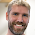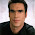## Sunday, June 10, 2012

### Social Network Analysis with neo4j: Graphs

I have been analyzing social networks and recently switched the database from standard relational to neo4j. The latter is much more appropriate for working with graphs and some basic insights about nodes and edges can be calculated in an instant.

The difference is obvious while dealing with big dataset. Below is an example SQL query for retrieving all connections among users in selected distance:

``` with recursive cluster (party, path, depth) ```
``` as ( select cast(@userId as character varying), cast(@userId as character varying), 1 ```
` union `
` ( `
` select (case `
` when this.party = amc.userA then amc.userB `
` when this.party = amc.userB then amc.userA `
` end), (this.path || '.' || (case `
` when this.party = amc.userA then amc.userB `
` when this.party = amc.userB then amc.userA `
` end)), this.depth + 1 `
` from cluster this, chat amc `
` where ((this.party = amc.userA and position(amc.userB in this.path) = 0) `
``` or (this.party = amc.userB and position(amc.userA in this.path) = 0)) AND this.depth < @depth + 1 ) ) ```
` select party, path `
` from cluster `
` where not exists ( `
` select * `
``` from cluster c2 where cluster.party = c2.party ```
` and ( `
` char_length(cluster.path) > char_length(c2.path)`
` or (char_length(cluster.path) = char_length(c2.path)) and (cluster.path > c2.path) `
` ) `
` ) `
``` order by party, path; ```

Running such query on database with several million users and connections takes very long time (talking in hours on proprietary PC).

Below is the Cypher query for neo4j database which counts all friends of friends (equivalent to above one in case of depth = 2)

`neo4j-sh (0)\$ start b = node:User(UserId='9F56478E6CAFB9CFF8C720C5DFC392C49495C582') MATCH (b) --(friend)--(friendoffriend) RETURN count(friendoffriend)`
`==> +-----------------------+`
`==> | count(friendoffriend) |`
`==> +-----------------------+`
`==> | 131457                |`
`==> +-----------------------+`
`==> 1 row, 635 ms`

The advantage in performance and simplicity is obvious.

## Running queries in neo4j console

Below are some more example Cypher queries for working with graphs. you can try out these and other on simple example network on this website.Graph Screenshot from neo4j Console

Find Neighbors

start a=node(*)
match (a)-->(b)
return a, b;
``` +-----------------------+ | a         | b         | +-----------------------+ | Node{} | Node{} | | Node{} | Node{} | | Node{} | Node{} | | Node{} | Node{} | | Node{} | Node{} | | Node{} | Node{} | | Node{} | Node{} | | Node{} | Node{} | | Node{} | Node{} | +-----------------------+ 9 rows 0 ms ``` Find Mutual Connections

``` start a=node(*), b=node(*) match (a)--(x)--(b) return a, b, x +-----------------------------------+ | a         | b         | x         | +-----------------------------------+ | Node{} | Node{} | Node{} | | Node{} | Node{} | Node{} | | Node{} | Node{} | Node{} | | Node{} | Node{} | Node{} | | Node{} | Node{} | Node{} | | Node{} | Node{} | Node{} | | Node{} | Node{} | Node{} | | Node{} | Node{} | Node{} | | Node{} | Node{} | Node{} | | Node{} | Node{} | Node{} | | Node{} | Node{} | Node{} | | Node{} | Node{} | Node{} | | Node{} | Node{} | Node{} | | Node{} | Node{} | Node{} | | Node{} | Node{} | Node{} | | Node{} | Node{} | Node{} | | Node{} | Node{} | Node{} | | Node{} | Node{} | Node{} | | Node{} | Node{} | Node{} | | Node{} | Node{} | Node{} | | Node{} | Node{} | Node{} | | Node{} | Node{} | Node{} | | Node{} | Node{} | Node{} | | Node{} | Node{} | Node{} | | Node{} | Node{} | Node{} | | Node{} | Node{} | Node{} | | Node{} | Node{} | Node{} | | Node{} | Node{} | Node{} | | Node{} | Node{} | Node{} | | Node{} | Node{} | Node{} | | Node{} | Node{} | Node{} | | Node{} | Node{} | Node{} | | Node{} | Node{} | Node{} | | Node{} | Node{} | Node{} | +-----------------------------------+ 34 rows 0 ms ```
Count Mutual Connections

``` start a=node(*), b=node(*) match (a)--(x)--(b) where id(a) < id(b) return a, b, count(distinct x) +-------------------------------------------+ | a         | b         | count(distinct x) | +-------------------------------------------+ | Node{} | Node{} | 1                 | | Node{} | Node{} | 2                 | | Node{} | Node{} | 1                 | | Node{} | Node{} | 1                 | | Node{} | Node{} | 1                 | | Node{} | Node{} | 1                 | | Node{} | Node{} | 1                 | | Node{} | Node{} | 2                 | | Node{} | Node{} | 1                 | | Node{} | Node{} | 1                 | | Node{} | Node{} | 1                 | | Node{} | Node{} | 1                 | | Node{} | Node{} | 1                 | | Node{} | Node{} | 1                 | | Node{} | Node{} | 1                 | +-------------------------------------------+ 15 rows 0 ms ```
Calculate Clustering Coefficient

``` start a = node(1) match (a)--(b) with a, b as neighbours match (a)--()-[r]-()--(a) where id(a) <> id(neighbours) and id(neighbours) <> 0 return count(distinct neighbours), count(distinct r) +------------------------------------------------+ | count(distinct neighbours) | count(distinct r) | +------------------------------------------------+ | 4                          | 1                 | +------------------------------------------------+ 1 row 0 ms ```
The clustering coefficient of a selected node is defined as probability that two randomly selected neighbors are connected to each other. So once having number of neighbors and number of mutual connections we can calculate:

1. The number of possible connections between two neighbors = n!/(2!(n-2)!) = 4!/(2!(4-2)!) = 24/4 = 6
where n is the number of neighbors n = 4

and the actual number of connections is 1,

therefore the clustering coefficient of node 1 is 1/6

References

Cypher Query Language

Networks, Crowds, and Markets: Reasoning About a Highly Connected World By David Easley and Jon Kleinberg

1.Cool post Niko!

I think you could omit "id(a) <> id(neighbours)" as these are bound to different variables and thus will not be the same.

Also, you probably can do the cluster coefficient calculation directly in the query as a last WITH part before returning (you can chain these). WDYT?

Also, if you provide a real setup in your console link (look at console.neo4j.org/usage.html to set up a graph) I would be thrilled to take this example into the Cypher Cookbook http://docs.neo4j.org/chunked/snapshot/cypher-cookbook.html ?

/peter

2.Thanks Peter!

The example network can be found here:
http://console.neo4j.org/?init=(A)%0A(B)%0A(C)%0A(D)%0A(E)%0A(F)%0A(G)%0A(0)-%5B%3AROOT%5D-%3E(A)%0A(A)-%5B%3AKNOWS%5D-%3E(B)%0A(A)-%5B%3AKNOWS%5D-%3E(C)%0A(A)-%5B%3AKNOWS%5D-%3E(D)%0A(A)-%5B%3AKNOWS%5D-%3E(E)%0A(B)-%5B%3AKNOWS%5D-%3E(F)%0A(B)-%5B%3AKNOWS%5D-%3E(G)%0A(C)-%5B%3AKNOWS%5D-%3E(D)%0A(E)-%5B%3AKNOWS%5D-%3E(F)&query=start%20a%3Dnode(1)%20match%20a-%5Br%3F%5D-other%20return%20a%2Ctype(r)%2C%20other%0A&version=

I just started out with Cypher and haven't found the way to calculate factorial with it. In the following days I will try to play with query optimization.

3.Hey Niko, this is really cool stuff! I'm mostly interested in the first example -- which DB is it you're using there? I'm experimenting a bit with SQL vs Cypher using HSQLDB, the code is over here: https://github.com/nawroth/community/blob/cyphersql/embedded-examples/src/main/java/org/neo4j/examples/CypherSql.java and the result will end up in the Neo4j Manual some day (it's mostly me and Peter working on that).

4.Hi Anders,

In the beginning I used Microsoft SQL and then switched to postgres.

To execute the queries in relational databases it took a long time (for example retrieving friends of friends of friends took at least half an hour on database with 40.000.000 connections)

The other problem is the complexity of queries - with neo4j and Cypher it is much easier as you can just "draw" the pattern you would like to extract from database.